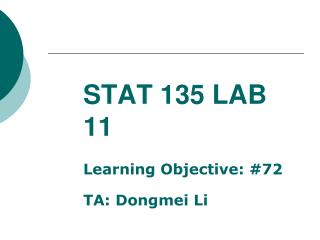DownloadDownload PresentationSTAT 135 LAB 11

# STAT 135 LAB 11

Download Presentation## STAT 135 LAB 11

- - - - - - - - - - - - - - - - - - - - - - - - - - - E N D - - - - - - - - - - - - - - - - - - - - - - - - - - -
##### Presentation Transcript

1. STAT 135 LAB 11 Learning Objective: #72 TA: Dongmei Li

2. Simulations in the Law of Average Lab (Lab 10) The number of red marbles in 38 trials: 16 16 19 17 26 13 with standard deviation =4.44597 Corresponding proportions (number of red marbles in 38 trials/38): 0.421053 0.421053 0.500000 0.447368 0.684211 0.342105 with standard deviation = 0.116999 The number of red marbles in 3800 trials: 1786 1792 1777 1826 1758 1822 with standard deviation=26.304 Corresponding proportions (number of red marbles in 3800 trials/3800): 0.470000 0.471579 0.467632 0.480526 0.462632 0.479474 with standard deviation = 0.00692210 • Conclusions: • When there are more trials, the proportions are likely to be more stable. • When there are more trials, the counts are likely to be more variable.

3. A Question Which is more likely to follow a normal distribution? • The weights of a randomly selected cartoon of large eggs from the grocer store shelf • The heights of all of the students in stat 135

4. Sampling distribution Take many samples (of same size) from the same population and calculate the sample mean or sample proportion from each sample. We can look at a distribution of the sample means or sample proportions. Sample mean and sample proportion are approximately normal if some conditions (see next slide) are satisfied.

5. Best normal approximation • When sample size is large ( ) • If and • If is close to and sample size is large

6. Learning Objective 72. The quality of the normal approximation improves with larger sample sizes and symmetry of the parent population.